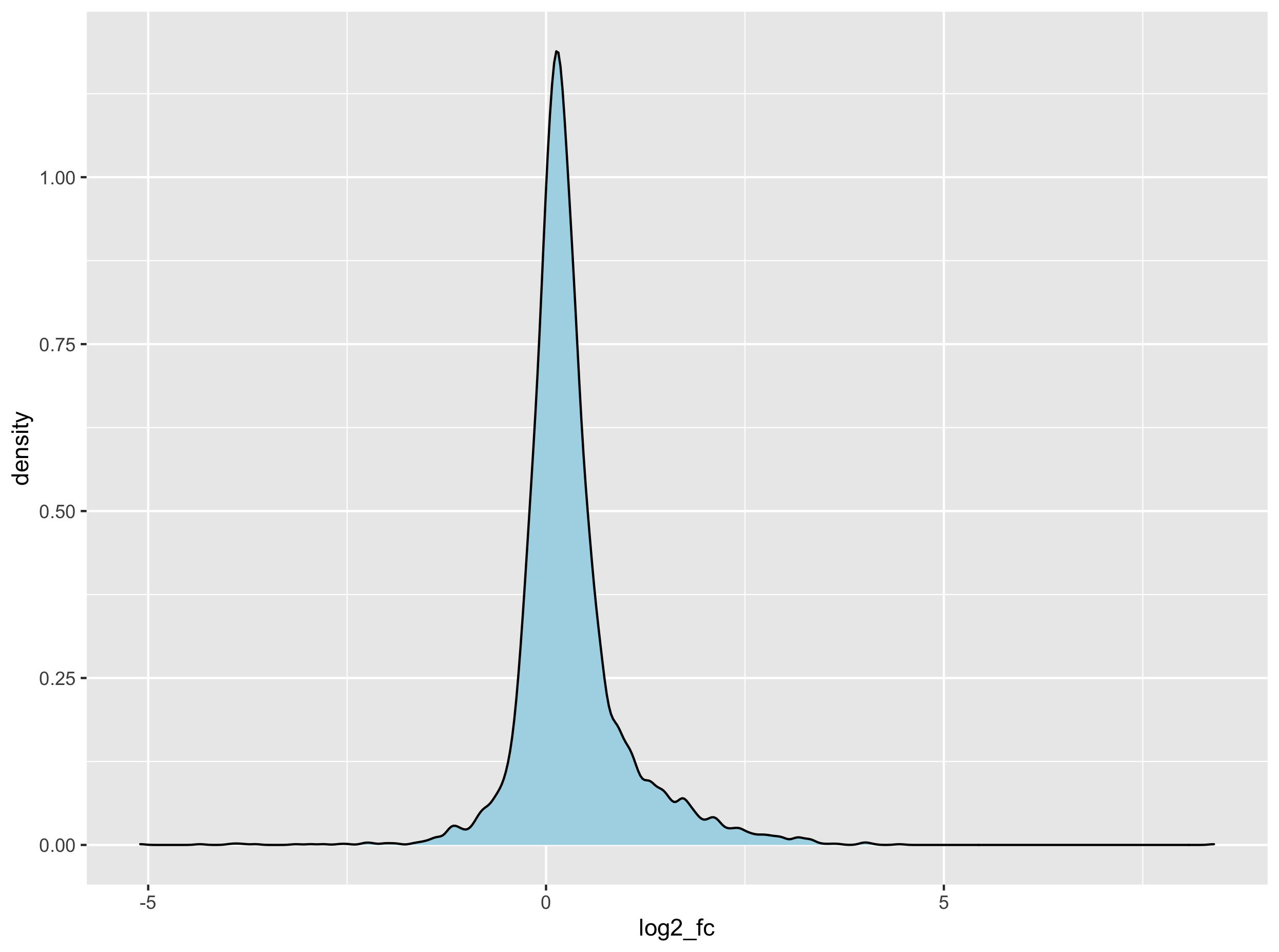This lesson is still being designed and assembled (Pre-Alpha version)

# Finding tissue-specific genes through feature engineering

## Overview

Teaching: 0 min
Exercises: 45 min
Questions
• How do I transform my variables to build more meaningful variables?

• What type of transformation and checks should I perform?

• How to extract my list of selected tissue-specific genes?

Objectives
• Understand how distances are calculated between two tissues based on their gene expression profile.

• Be able to name a few different clustering methods.

# 1. Introduction

There is an alternative perhaps more intuitive method to find tissue-specific genes. Actually this is the method used by Ahn and co-authors in their publication.

Here are the different steps that will be undertaken:

1. Create a dataframe that only contains the gene TPM values for our tissue of interest (e.g. subcutaneous adipose tissue)
2. Create a dataframe that contains all other tissues gene TPM values.
3. Compute the median TPM value per gene for the other tissues.
4. Join the two dataframes.
5. Calculate the subcutaneous adipose versus _all other tissues__ fold changes.
6. Converting this fold change to a $$log_{2}$$ fold change to normalise the distribution of the fold changes.
7. Perform a $$Z$$-test analysis to compute p-values.
8. Extract the genes with the highest and most significant $$log_{2}$$ fold changes.

# 2. Feature engineering

Here, feature engineering refers to the grouping of the other tissues under a new variable called “other_tissues”. This will turn our complex multivariate analysis (see PCA and clustering episodes) into a simpler univariate analysis.

## 2.1 Filtering genes with low expression values

Import or re-import the data first.

df_expr <- read.delim(file = "data/GTEx_Analysis_2016-01-15_v7_RNASeQCv1.1.8_gene_median_tpm.tsv",
stringsAsFactors = FALSE,
check.names = FALSE)

df_expr_tidy <- df_expr %>%
select(- Description) %>%
pivot_longer(- gene_id, names_to = "tissue", values_to = "tpm")

threshold = df_expr_tidy %>%
group_by(gene_id) %>%
summarise(median_tpm = median(tpm)) %>%
with(., round(x = quantile(x = median_tpm, probs = 0.9)))

genes_selected =
df_expr_tidy %>%
group_by(gene_id) %>%
summarise(median_tpm = median(tpm)) %>%
ungroup() %>%
filter(median_tpm > threshold) %>%
dplyr::pull(gene_id)

df_expr_tidy_filtered <- filter(df_expr_tidy, gene_id %in% genes_selected)


## 2.2 Creating the adipose and other tissues dataframes

# extract gene TPM value in "Adipose - Subcutaneous"
filter(tissue == "Adipose - Subcutaneous") %>%
select(gene_id, tpm) %>%

# calculate median TPM value in all other tissues
all_other_tissues_gene_expression <-
df_expr_tidy_filtered %>%
filter(tissue != "Adipose - Subcutaneous") %>%
group_by(gene_id) %>%
summarise(other_tissues_median_tpm = median(tpm))


## 2.3 Merge dataframes and calculate log2 fold changes

## Merge the two dataframes
## Calculate a fold change
y = all_other_tissues_gene_expression,
by = "gene_id") %>%
mutate(fc = adipose_tpm / other_tissues_median_tpm) %>%
mutate(log2_fc = log2(fc))


# 3. Compute $$Z$$-tests and extract adipose-specific genes

Since our sample size is relatively large (> 50), we can perform a $$Z$$-test analysis to compute the probability

## 3.1 Normality test

# normal distribution of log2FC?
geom_density()

# calculate Z-score
mean_of_log2fc <- with(data = adipose_vs_other_tissues, mean(log2_fc))
sd_of_log2fc <- with(data = adipose_vs_other_tissues, sd(log2_fc))

# test for normality
ks.test(x = adipose_vs_other_tissues$log2_fc, y = "pnorm", mean = mean_of_log2fc, sd = sd_of_log2fc)Distrinbution of gene log2 fold changes The Kolmogorov–Smirnov test that compares the observed distribution of the $$log_{2}$$ fold change to its theoretical normal distribution (based on its observed mean and variance). The null hypothesis stipulates that the observed distribution are drawn from its theoretical normal distribution. A large $$D$$ score will convert to a small p-value therefore suggesting that the distribution significantly deviates from its theoretical normal distribution. One-sample Kolmogorov-Smirnov test data: adipose_vs_other_tissues$log2_fc
D = 0.14537, p-value < 2.2e-16
alternative hypothesis: two-sided



Although our data is not strictly normal sensu stricto, we will assume it is for demonstration purposes and since a high $$log_{2}$$ will nethertheless indicate a high expression in subcutaneous adipose tissue.

## 3.2 $$Z$$-test

The $$Z$$-test can be used to test whether an observed $$log_{2}$$ fold change is significantly different from the population average $$log_{2}$$ fold changes. To perform this analysis, we can compute the $$Z$$-score for each of the $$log_{2}$$. In turn, this allows to convert this score to a probability for each of the individual gene fold change.

adipose_vs_other_tissues$zscore <- map_dbl( adipose_vs_other_tissues$log2_fc,
function(x) (x - mean_of_log2fc) / sd_of_log2fc
)


## 3.3 Compute p-values and extract final list

Here, p-values will be extracted using a one-tailed p-value since we want log2 fold changes higher than

# filter fc > 0 + calculate one-sided p-value
filter(log2_fc > 0) %>%                   # FC superior to 1
mutate(pval = 1 - pnorm(zscore)) %>%      # one-tailed p-value
filter(pval < 0.01) %>%
arrange(desc(log2_fc))



This yields the top 10 genes:

# A tibble: 10 x 7
gene_id           adipose_tpm other_tissues_median_tpm    fc log2_fc zscore     pval
<chr>                   <dbl>                    <dbl> <dbl>   <dbl>  <dbl>    <dbl>
1 ENSG00000170323.4       5946.                     17.7 336.     8.39  12.1  0.
2 ENSG00000196616.8       1302                      60.0  21.7    4.44   6.16 3.54e-10
3 ENSG00000165507.8       1019                      59.8  17.0    4.09   5.64 8.47e- 9
4 ENSG00000197766.3       2090.                    129.   16.2    4.02   5.53 1.60e- 8
5 ENSG00000131471.2        320.                     19.9  16.0    4.00   5.51 1.79e- 8
6 ENSG00000174807.3        374.                     23.8  15.8    3.98   5.47 2.19e- 8
7 ENSG00000167772.7        221.                     17.3  12.7    3.67   5.02 2.65e- 7
8 ENSG00000147872.5        326.                     27.0  12.1    3.59   4.90 4.77e- 7
9 ENSG00000145824.8        518.                     46.3  11.2    3.48   4.73 1.10e- 6
10 ENSG00000008394.8        168.                     16.0  10.5    3.39   4.59 2.17e- 6


In total, you should have 195 genes with a significant positive fold change related to subcutaneous adipose tissue.

## Exercise 1

Navigate to the GTEx portal and search for additional information about these genes.

## Exercise 2

Heatmap revived! Using the list of subcutaneous adipose-related genes:

1. Filter the original dataset to keep only the 195 adipose-related genes.
2. Convert to matrix and scale the matrix so that gene expression values become comparable.
3. Build a heatmap using your own clustering method (or the default one from pheatmap())
4. Now try to keep only the top 20 genes with the highest fold change and rebuild your heatmap.

## Key Points

• Sometimes, creating a new variable is a necessary step to find interesting leads in a dataset.

• Data transformation that converts a distribution to a normal one can benefit to one’s analysis.